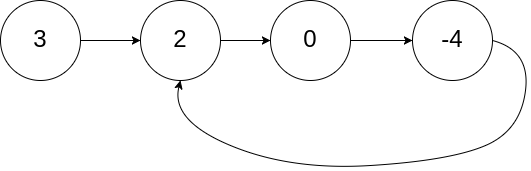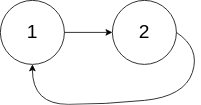20200503

          5
/ \
4   8
/   / \
11  13  4
/  \      \
7    2      1

class Solution {
public:
bool hasPathSum(TreeNode* root, int sum) {
if(!root) return false;
sum = sum - root->val;
if(!root->left && !root->right && sum == 0) return true;
return hasPathSum(root->left,sum) || hasPathSum(root->right,sum);
}
};[
,
[1,1],
[1,2,1],
[1,3,3,1],
[1,4,6,4,1]
]

class Solution {
public:
vector<vector<int>> generate(int numRows) {
vector<vector<int>> ans(numRows);
if(numRows == 0)    return ans;
for(int i = 0; i < numRows; ++ i )
{
for(int j = 0; j <= i; ++ j)
{
if(j == 0 || j == i)
ans[i].push_back(1);
else
ans[i].push_back(ans[i-1][j-1] + ans[i-1][j]);
}
}
return ans;
}
};


20200504class Solution {
public:
vector<int> getRow(int rowIndex) {
vector<vector<int>> ans(rowIndex+1);
for(int i = 0; i <= rowIndex; ++ i )
{
for(int j = 0; j <= i; ++ j)
{
if(j == 0 || j == i)
ans[i].push_back(1);
else
ans[i].push_back(ans[i-1][j-1] + ans[i-1][j]);
}
}
return ans[rowIndex];
}
};


class Solution {
public:
int maxProfit(vector<int>& prices) {
int max_val = 0;
int min_val = INT_MAX;
for(int i = 0;i<prices.size();++i)
{
min_val = min(min_val,prices[i]);
max_val = max(max_val,prices[i]-min_val);
}
return max_val;
}
};


20200505

class Solution {
public:
int maxProfit(vector<int>& prices) {
int profit = 0;
for(int i=0;i < prices.size()-1;++i)
profit += max(prices[i+1]-prices[i],0);
return profit;
}
};


class Solution {
public:
bool isPalindrome(string s) {
if(s.size()<=1) return true;
int i = 0,j=s.size()-1;
while(i<j)
{
while(i<j && !isalnum(s[i]))
i++;
while(i<j && !isalnum(s[j]))
j--;
if(tolower(s[i++])!=tolower(s[j--]))
return false;
}
return true;
}
};


20200506

XOR 运算满足交换律和结合律： a⊕b⊕a = (a⊕a)⊕b = 0⊕b = b

class Solution {
public:
int singleNumber(vector<int>& nums) {
int res = 0;
for(int i = 0;i < nums.size();++i)
res ^= nums[i];
return res;
}
};


20200507/**
* struct ListNode {
*     int val;
*     ListNode *next;
*     ListNode(int x) : val(x), next(NULL) {}
* };
*/
class Solution {
public:
while(faster!=NULL && faster->next!=NULL)
{
faster = faster->next->next;
lower = lower->next;
if(faster == lower)
return true;
}
return false;
}
};


20200509class Solution {
public:
while(A)
{
while(B)
{
if(A == B)
return A;
B = B->next;
}
A = A->next;
}
return NULL;
}
};


20200511

class Solution {
public:
vector<int> twoSum(vector<int>& numbers, int target) {
int i = 0;
int j = numbers.size() - 1;
while(i < j)
{
int sum = numbers[i] + numbers[j];
if(sum < target)
i++;
else if(sum > target)
j--;
else
return vector<int>{i+1,j+1};
}
return vector<int>{-1,-1};
}
};


1 -> A
2 -> B
3 -> C
...
26 -> Z
27 -> AA
28 -> AB
...

class Solution {
public:
string convertToTitle(int n) {
string ans = "";
while(n > 0)
{
n-=1;
ans.push_back('A' + (n%26));
n /= 26;
}
reverse(ans.begin(),ans.end());
return ans;
}
};
Last modification：May 29th, 2020 at 11:08 pm